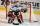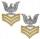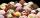# Hockey game

In the hockey game was made 6 goals. Czech played against Finland. Czechs won 4:2. In what order to fall goals? How many game sequence was possible during the game?

Result

n =  15

#### Solution:Leave us a comment of example and its solution (i.e. if it is still somewhat unclear...):Be the first to comment!#### To solve this example are needed these knowledge from mathematics:

See also our combinations with repetition calculator. Would you like to compute count of combinations?

## Next similar examples:

1. HockeyHockey match ended 8:2. How many different matches could be?
2. ChordsHow many 4-tones chords (chord = at the same time sounding different tones) is possible to play within 7 tones?
3. TeamsHow many ways can divide 16 players into two teams of 8 member?
4. BlocksThere are 9 interactive basic building blocks of an organization. How many two-blocks combinations are there?
5. Calculation of CNCalculate: ?
6. First classThe shipment contains 40 items. 36 are first grade, 4 are defective. How many ways can select 5 items, so that it is no more than one defective?
7. DivisionDivision has 18 members: 10 girls and 6 boys, 2 leaders. How many different patrols can be created, if one patrol is 2 boys, 3 girls and 1 leader?
8. The confectioneryThe confectionery sold 5 kinds of ice cream. In how many ways can I buy 3 kinds if order of ice creams does not matter?
9. Count of trianglesGiven a square ABCD and on each side 8 internal points. Determine the number of triangles with vertices at these points.
10. PIN - codesHow many five-digit PIN - code can we create using the even numbers?On the menu are 12 kinds of meal. How many ways can we choose four different meals into the daily menu?A fish tank at a pet store has 8 zebra fish. In how many different ways can George choose 2 zebra fish to buy?The class is 21 students. How many ways can choose two to examination?We want to prove the sentence: If the natural number n is divisible by six, then n is divisible by three. From what assumption we started?How many different triads can be selected from the group 43 students?A student is to answer 8 out of 10 questions on the exam. a) find the number n of ways the student can choose 8 out of 10 questions b) find n if the student must answer the first three questions c) How many if he must answer at least 4 of the first 5 queIn the class are 20 pupils. How many opportunities have the teacher if he wants choose two pupils randomly who will weeklies?